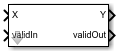# Divide by Constant HDL Optimized

Divide input by a constant and round to integer and generate optimized HDL code

• Library:
• Fixed-Point Designer HDL Support / Math Operations

•## Description

The Divide by Constant HDL Optimized block outputs the result of dividing the input by a constant and rounds the result to an integer using the specified rounding method using an HDL-optimized architecture with cycle-true latency.

The Divide by Constant HDL Optimized block uses an algorithm that is functionally similar to the Granlund-Montgomery-Warren Method. The division operation is computed via a multiplication by inverse, which generally results in better performance on embedded systems.

## Ports

### Input

expand all

Dividend, specified as a real scalar.

Data Types: `single` | `double` | `int8` | `int16` | `int32` | `uint8` | `uint16` | `uint32` | `Boolean` | `fixed point`

Whether input is valid, specified as a Boolean scalar. This control signal indicates when the data from the X input port is valid. When this value is `1` (`true`), the block captures the value on the X input port. When this value is `0` (`false`), the block ignores the input samples.

Data Types: `Boolean`

### Output

expand all

Result of division and round operation, returned as a scalar.

Data Types: `single` | `double` | `int8` | `int16` | `int32` | `uint8` | `uint16` | `uint32` | `Boolean` | `fixed point`

Whether the output data is valid, returned as a Boolean scalar. When the value of this control signal is `1` (`true`), the block has successfully computed the output Y. When this value is `0` (`false`), the output data is not valid.

Data Types: `Boolean`

## Parameters

expand all

Divisor, specified as a positive, real-valued, finite scalar.

#### Programmatic Use

 Block Parameter: `Denominator` Type: character vector Values: MATLAB® expression that evaluates to a positive, real-valued, finite fixed point or numeric value Default: `'10'`

Data Types: `single` | `double` | `int8` | `int16` | `int32` | `int64` | `uint8` | `uint16` | `uint32` | `uint64` | `Boolean` | `fixed point`

Rounding method to use, specified as one of these values:

• `Floor` — Round to nearest integer in the direction of negative infinity.

• `Ceiling` — Round to nearest integer in the direction of positive infinity.

• `Nearest` — Round to the nearest integer. Ties are rounded to the nearest integer in the direction of positive infinity.

• `Zero` — Round to the nearest integer in the direction of zero.

• `Convergent` — Round to the nearest integer. Ties are rounded to the nearest even integer.

#### Programmatic Use

 Block Parameter: `RndMeth` Type: character vector Values: `'Floor'` | `'Ceiling'` | `'Nearest'` | `'Zero'` | `'Convergent'` Default: `'Floor'`

## Tips

The blocks Divide by Constant HDL Optimized, Real Divide HDL Optimized, and Complex Divide HDL Optimized all perform the division operation and generate optimized HDL code.

• Real Divide HDL Optimized and Complex Divide HDL Optimized are based on a CORIDC algorithm. These blocks accept a wide variety of inputs, but will result in greater latency.

• Divide by Constant HDL Optimized accepts only real inputs and a constant divisor. Use of this block consumes DSP slices, but will complete the division operation in fewer cycles and at a higher clock rate.

## Extended Capabilities

### Topics

Introduced in R2021a

## Support평가판 신청